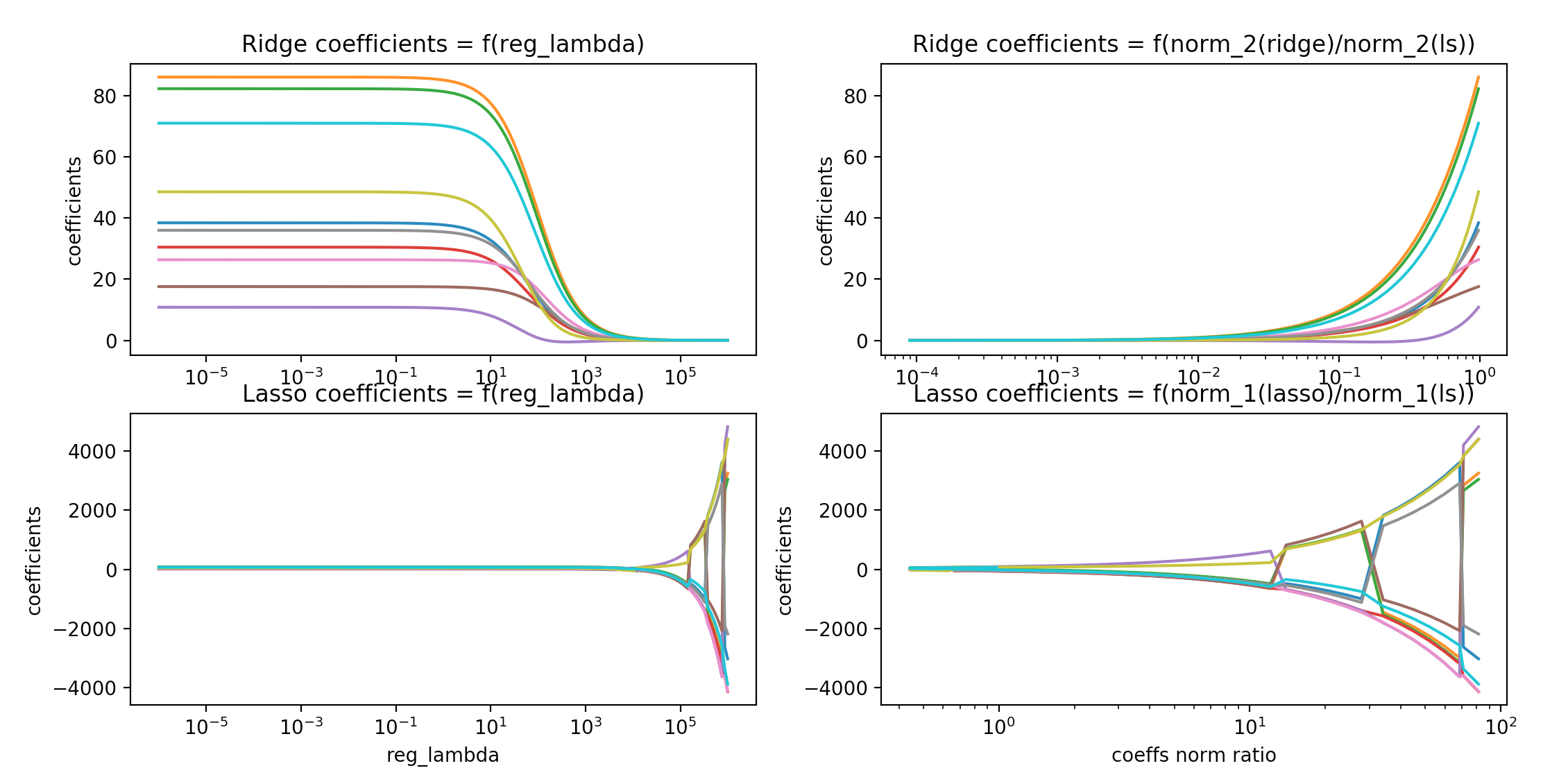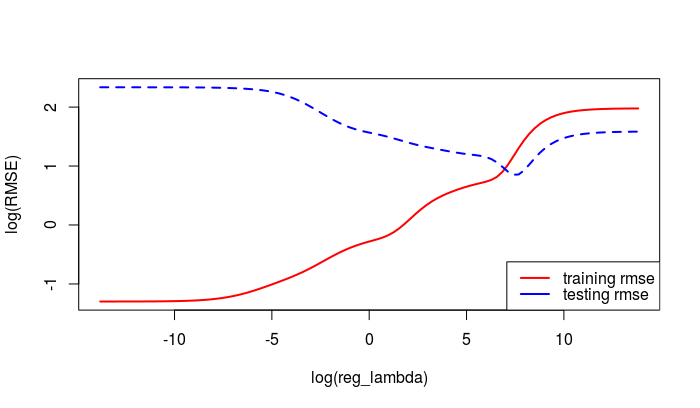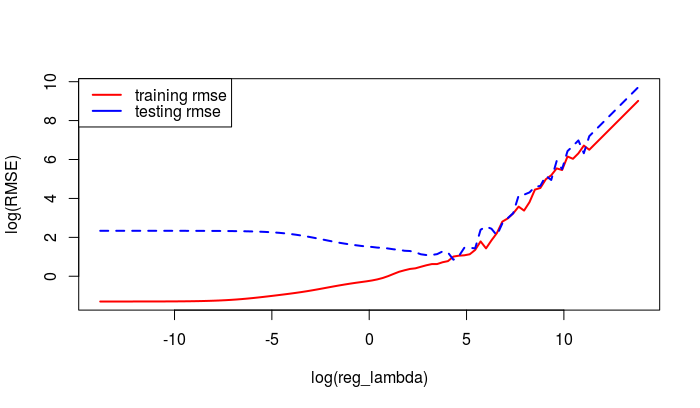This post is about LSBoost, an Explainable ‘AI’ algorithm which uses Gradient Boosted randomized networks for pattern recognition. As we’ve discussed it last week LSBoost is a cousin of GFAGBM’s LS_Boost. In LSBoost, more specifically, the so called weak learners from LS_Boost are based on randomized neural networks’ components and variants of Least Squares regression models.

I’ve already presented some promising examples of use of LSBoost based on Ridge Regression weak learners. In mlsauce’s version 0.7.1, the Lasso can also be used as an alternative ingredient to the weak learners. Here is a comparison of the regression coefficients obtained by using mlsauce’s implementation of Ridge regression and the Lasso:R example: LSBoostRegressor with Ridge regression and the Lasso

The following example is about training set error vs testing set error, as a function of the regularization parameter, both for Ridge regression and Lasso-based weak learners.

Packages and data

# 0 - Packages and data -------------------------------------------------------

library(devtools)
devtools::install_github("thierrymoudiki/mlsauce/R-package")
library(mlsauce)
library(datasets)

print(summary(datasets::mtcars))

X <- as.matrix(datasets::mtcars[, -1])
y <- as.integer(datasets::mtcars[, 1])

n <- dim(X)
p <- dim(X)
set.seed(21341)
train_index <- sample(x = 1:n, size = floor(0.8*n), replace = TRUE)
test_index <- -train_index
X_train <- as.matrix(X[train_index, ])
y_train <- as.double(y[train_index])
X_test <- as.matrix(X[test_index, ])
y_test <- as.double(y[test_index])

LSBoost using Ridge regression

# 1 - Ridge -------------------------------------------------------------------

obj <- mlsauce::LSBoostRegressor() # default h is Ridge
print(obj$get_params()) n_lambdas <- 100 lambdas <- 10**seq(from=-6, to=6, length.out = n_lambdas) rmse_matrix <- matrix(NA, nrow = 2, ncol = n_lambdas) rownames(rmse_matrix) <- c("training rmse", "testing rmse") for (j in 1:n_lambdas) { obj$set_params(reg_lambda = lambdas[j])
obj$fit(X_train, y_train) rmse_matrix[, j] <- c(sqrt(mean((obj$predict(X_train) - y_train)**2)),
sqrt(mean((obj$predict(X_test) - y_test)**2))) }LSBoost using the Lasso # 2 - Lasso ------------------------------------------------------------------- obj <- mlsauce::LSBoostRegressor(solver = "lasso") print(obj$get_params())

n_lambdas <- 100
lambdas <- 10**seq(from=-6, to=6,
length.out = n_lambdas)
rmse_matrix2 <- matrix(NA, nrow = 2, ncol = n_lambdas)
rownames(rmse_matrix2) <- c("training rmse", "testing rmse")

for (j in 1:n_lambdas)
{
obj$set_params(reg_lambda = lambdas[j]) obj$fit(X_train, y_train)
rmse_matrix2[, j] <- c(sqrt(mean((obj$predict(X_train) - y_train)**2)), sqrt(mean((obj$predict(X_test) - y_test)**2)))
}R session info

> print(session_info())
Session info ─────────────────────────────────────────────────────────────
setting  value
version  R version 4.0.2 (2020-06-22)
os       Ubuntu 16.04.6 LTS
system   x86_64, linux-gnu
ui       RStudio
language (EN)
collate  C.UTF-8
ctype    C.UTF-8
tz       Etc/UTC
date     2020-07-31

Packages ─────────────────────────────────────────────────────────────────
package     * version date       lib source
assertthat    0.2.1   2019-03-21  RSPM (R 4.0.2)
backports     1.1.8   2020-06-17  RSPM (R 4.0.2)
callr         3.4.3   2020-03-28  RSPM (R 4.0.2)
cli           2.0.2   2020-02-28  RSPM (R 4.0.2)
crayon        1.3.4   2017-09-16  RSPM (R 4.0.2)
curl          4.3     2019-12-02  RSPM (R 4.0.2)
desc          1.2.0   2018-05-01  RSPM (R 4.0.2)
devtools    * 2.3.1   2020-07-21  RSPM (R 4.0.2)
digest        0.6.25  2020-02-23  RSPM (R 4.0.2)
ellipsis      0.3.1   2020-05-15  RSPM (R 4.0.2)
fansi         0.4.1   2020-01-08  RSPM (R 4.0.2)
fs            1.4.2   2020-06-30  RSPM (R 4.0.2)
glue          1.4.1   2020-05-13  RSPM (R 4.0.2)
jsonlite      1.7.0   2020-06-25  RSPM (R 4.0.2)
lattice       0.20-41 2020-04-02  CRAN (R 4.0.2)
magrittr      1.5     2014-11-22  RSPM (R 4.0.2)
Matrix        1.2-18  2019-11-27  CRAN (R 4.0.2)
memoise       1.1.0   2017-04-21  RSPM (R 4.0.2)
mlsauce     * 0.7.1   2020-07-31  Github (thierrymoudiki/mlsauce@68e391a)
pkgbuild      1.1.0   2020-07-13  RSPM (R 4.0.2)
pkgload       1.1.0   2020-05-29  RSPM (R 4.0.2)
prettyunits   1.1.1   2020-01-24  RSPM (R 4.0.2)
processx      3.4.3   2020-07-05  RSPM (R 4.0.2)
ps            1.3.3   2020-05-08  RSPM (R 4.0.2)
R6            2.4.1   2019-11-12  RSPM (R 4.0.2)
rappdirs      0.3.1   2016-03-28  RSPM (R 4.0.2)
Rcpp          1.0.5   2020-07-06  RSPM (R 4.0.2)
remotes       2.2.0   2020-07-21  RSPM (R 4.0.2)
reticulate    1.16    2020-05-27  RSPM (R 4.0.2)
rlang         0.4.7   2020-07-09  RSPM (R 4.0.2)
rprojroot     1.3-2   2018-01-03  RSPM (R 4.0.2)
rstudioapi    0.11    2020-02-07  RSPM (R 4.0.2)
sessioninfo   1.1.1   2018-11-05  RSPM (R 4.0.2)
testthat      2.3.2   2020-03-02  RSPM (R 4.0.2)
usethis     * 1.6.1   2020-04-29  RSPM (R 4.0.2)
withr         2.2.0   2020-04-20  RSPM (R 4.0.2)

 /home/rstudio-user/R/x86_64-pc-linux-gnu-library/4.0
 /opt/R/4.0.2/lib/R/library

No post in August